SLOS369G July   2002  – October 2015

PRODUCTION DATA.

1. Features
2. Applications
3. Description
4. Revision History
5. Device Comparison Table
6. Pin Configuration and Functions
7. Specifications
8. Parameter Measurement Information
9. Detailed Description
1. 9.1 Overview
2. 9.2 Functional Block Diagram
3. 9.3 Feature Description
4. 9.4 Device Functional Modes
10. 10Application and Implementation
1. 10.1 Application Information
2. 10.2 Typical Applications
1. 10.2.1 TPA2005D1 with Differential Input
2. 10.2.2 TPA2005D1 with Differential Input and Input Capacitors
1. 10.2.2.1 Design Requirements
2. 10.2.2.2 Detailed Design Procedure
3. 10.2.3 TPA2005D1 with Single-Ended Input
11. 11Power Supply Recommendations
12. 12Layout
1. 12.1 Layout Guidelines
2. 12.2 Layout Examples
13. 13Device and Documentation Support
14. 14Mechanical, Packaging, and Orderable Information

#### Package Options

Refer to the PDF data sheet for device specific package drawings

• DGN|8
• DRB|8
• DRB|8
• DGN|8

## 9 Detailed Description

### 9.1 Overview

The TPA2005D1 is a high-efficiency filter-free Class-D audio amplifier capable of delivering up to 1.4 W into 8-Ω loads with 5-V power supply. The fully-differential design of this amplifier avoids the usage of bypass capacitors and the improved CMRR eliminates the usage of input-coupling capacitors. This makes the device size a perfect choice for small, portable applications as only three external components are required.

The advanced modulation used in the TPA2005D1 PWM output stage eliminates the need for an output filter.

### 9.2 Functional Block Diagram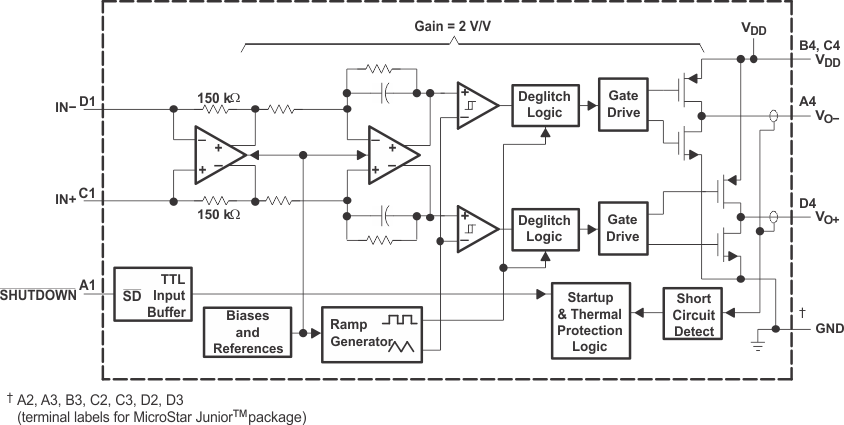### 9.3 Feature Description

#### 9.3.1 Fully Differential Amplifier

The TPA2005D1 is a fully differential amplifier with differential inputs and outputs. The fully differential amplifier consists of a differential amplifier and a common-mode amplifier. The differential amplifier ensures that the amplifier outputs a differential voltage on the output that is equal to the differential input times the gain. The common-mode feedback ensures that the common-mode voltage at the output is biased around VDD/2 regardless of the common-mode voltage at the input. The fully differential TPA2005D1 can still be used with a single-ended input; however, the TPA2005D1 should be used with differential inputs when in a noisy environment, like a wireless handset, to ensure maximum noise rejection.

#### 9.3.1.1 Advantages of Fully Differential Amplifiers

• Input-coupling capacitors not required:
• The fully differential amplifier allows the inputs to be biased at voltage other than mid-supply. For example, if a codec has a midsupply lower than the midsupply of the TPA2005D1, the common-mode feedback circuit will adjust, and the TPA2005D1 outputs will still be biased at midsupply of the TPA2005D1. The inputs of the TPA2005D1 can be biased from 0.5 V to VDD - 0.8 V. If the inputs are biased outside of that range, input-coupling capacitors are required.
• Midsupply bypass capacitor, C(BYPASS), not required:
• The fully differential amplifier does not require a bypass capacitor. This is because any shift in the midsupply affects both positive and negative channels equally and cancels at the differential output.
• Better RF-immunity:
• GSM handsets save power by turning on and shutting off the RF transmitter at a rate of 217 Hz. The transmitted signal is picked-up on input and output traces. The fully differential amplifier cancels the signal much better than the typical audio amplifier.

#### 9.3.2 Efficiency and Thermal Information

The maximum ambient temperature depends on the heat-sinking ability of the PCB system. The derating factor for the 2,5-mm x 2,5-mm MicroStar Junior package is shown in the dissipation rating table. Converting this to θJA:

Equation 1.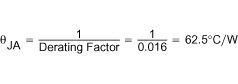Given θJA of 62.5°C/W, the maximum allowable junction temperature of 150°C, and the maximum internal dissipation of 0.2 W (worst case 5-V supply), the maximum ambient temperature can be calculated with equation Equation 2.

Equation 2.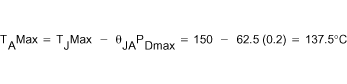Equation Equation 2 shows that the calculated maximum ambient temperature is 137.5°C at maximum power dissipation with a 5-V supply; however, the maximum ambient temperature of the package is limited to 85°C. Because of the efficiency of the TPA2005D1, it can be operated under all conditions to an ambient temperature of 85°C. The TPA2005D1 is designed with thermal protection that turns the device off when the junction temperature surpasses 150°C to prevent damage to the IC. Also, using speakers more resistive than 8-Ω dramatically increases the thermal performance by reducing the output current and increasing the efficiency of the amplifier.

#### 9.3.3 Eliminating the Output Filter with the TPA2005D1

This section focuses on why the user can eliminate the output filter with the TPA2005D1.

#### 9.3.3.1 Effect on Audio

The class-D amplifier outputs a pulse-width modulated (PWM) square wave, which is the sum of the switching waveform and the amplified input audio signal. The human ear acts as a band-pass filter such that only the frequencies between approximately 20 Hz and 20 kHz are passed. The switching frequency components are much greater than 20 kHz, so the only signal heard is the amplified input audio signal.

#### 9.3.3.2 Traditional Class-D Modulation Scheme

The traditional class-D modulation scheme, which is used in the TPA005Dxx family, has a differential output where each output is 180 degrees out of phase and changes from ground to the supply voltage, VDD. Therefore, the differential pre-filtered output varies between positive and negative VDD, where filtered 50% duty cycle yields 0 volts across the load. The traditional class-D modulation scheme with voltage and current waveforms is shown in Figure 33. Note that even at an average of 0 volts across the load (50% duty cycle), the current to the load is high causing a high loss and thus causing a high supply current.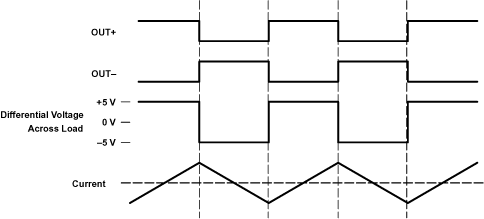Figure 33. Traditional Class-D Modulation Scheme's Output Voltage and Current Waveforms Into an Inductive Load With no Input

#### 9.3.3.3 TPA2005D1 Modulation Scheme

The TPA2005D1 uses a modulation scheme that still has each output switching from 0 to the supply voltage. However, OUT+ and OUT- are now in phase with each other with no input. The duty cycle of OUT+ is greater than 50% and OUT- is less than 50% for positive voltages. The duty cycle of OUT+ is less than 50% and OUT- is greater than 50% for negative voltages. The voltage across the load sits at 0 volts throughout most of the switching period greatly reducing the switching current, which reduces any I2R losses in the load.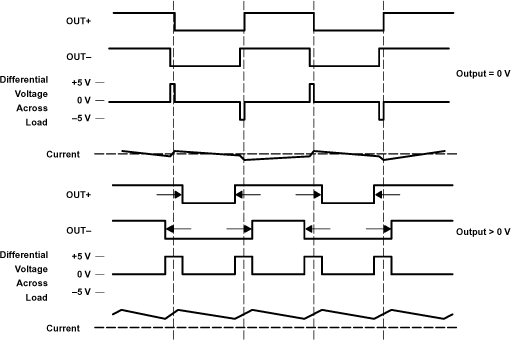Figure 34. The TPA2005D1 Output Voltage and Current Waveforms Into an Inductive Load

#### 9.3.3.4 Efficiency: Why You Must Use a Filter With the Traditional Class-D Modulation Scheme

The main reason that the traditional class-D amplifier needs an output filter is that the switching waveform results in maximum current flow. This causes more loss in the load, which causes lower efficiency. The ripple current is large for the traditional modulation scheme because the ripple current is proportional to voltage multiplied by the time at that voltage. The differential voltage swing is 2 × VDD and the time at each voltage is half the period for the traditional modulation scheme. An ideal LC filter is needed to store the ripple current from each half cycle for the next half cycle, while any resistance causes power dissipation. The speaker is both resistive and reactive, whereas an LC filter is almost purely reactive.

The TPA2005D1 modulation scheme has little loss in the load without a filter because the pulses are short and the change in voltage is VDD instead of 2 × VDD. As the output power increases, the pulses widen making the ripple current larger. Ripple current could be filtered with an LC filter for increased efficiency, but for most applications the filter is not needed.

An LC filter with a cutoff frequency less than the class-D switching frequency allows the switching current to flow through the filter instead of the load. The filter has less resistance than the speaker that results in less power dissipated, which increases efficiency.

#### 9.3.3.5 Effects of Applying a Square Wave Into a Speaker

If the amplitude of a square wave is high enough and the frequency of the square wave is within the bandwidth of the speaker, a square wave could cause the voice coil to jump out of the air gap and/or scar the voice coil. A 250-kHz switching frequency, however, is not significant because the speaker cone movement is proportional to 1/f2 for frequencies beyond the audio band. Therefore, the amount of cone movement at the switching frequency is small. However, damage could occur to the speaker if the voice coil is not designed to handle the additional power. To size the speaker for added power, the ripple current dissipated in the load needs to be calculated by subtracting the theoretical supplied power, PSUP THEORETICAL, from the actual supply power, PSUP, at maximum output power, POUT. The switching power dissipated in the speaker is the inverse of the measured efficiency,η MEASURED, minus the theoretical efficiency,η THEORETICAL.

Equation 3.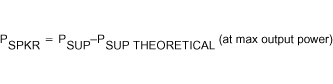Equation 4.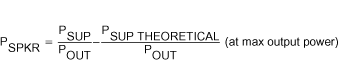Equation 5.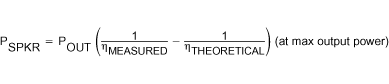Equation 6.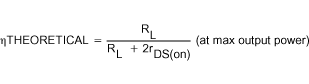The maximum efficiency of the TPA2005D1 with a 3.6 V supply and an 8-Ω load is 86% from equation Equation 6. Using equation Equation 5 with the efficiency at maximum power (84%), we see that there is an additional 17 mW dissipated in the speaker. The added power dissipated in the speaker is not an issue as long as it is taken into account when choosing the speaker.

#### 9.3.3.6 When to Use an Output Filter

Design the TPA2005D1 without an output filter if the traces from amplifier to speaker are short. The TPA2005D1 passed FCC and CE radiated emissions with no shielding with speaker trace wires 100 mm long or less. Wireless handsets and PDAs are great applications for class-D without a filter.

A ferrite bead filter can often be used if the design is failing radiated emissions without an LC filter, and the frequency sensitive circuit is greater than 1 MHz. This is good for circuits that just have to pass FCC and CE because FCC and CE only test radiated emissions greater than 30 MHz. If choosing a ferrite bead, choose one with high impedance at high frequencies, but low impedance at low frequencies.

Use an LC output filter if there are low frequency (< 1 MHz) EMI sensitive circuits and/or there are long leads from amplifier to speaker.

Figure 35 and Figure 36 show typical ferrite bead and LC output filters.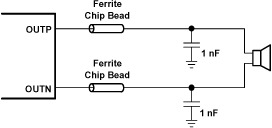Figure 35. Typical Ferrite Chip Bead Filter (Chip bead example: NEC/Tokin: N2012ZPS121)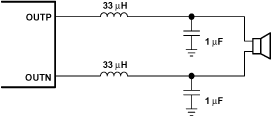Figure 36. Typical LC Output Filter, Cutoff Frequency of 27 kHz

#### 9.3.4 Thermal and Short-Circuit Protection

The TPA2005D1 features thermal and short-circuit protection. When the protection circuit is triggered, the device will enter in shutdown mode, setting the outputs of the device into high impedance. Thermal protection turns the device off when the junction temperature surpasses 150°C to prevent damage to the IC.

### 9.4 Device Functional Modes

#### 9.4.1 Summing Input Signals with the TPA2005D1

Most wireless phones or PDAs need to sum signals at the audio power amplifier or just have two signal sources that need separate gain. The TPA2005D1 makes it easy to sum signals or use separate signal sources with different gains. Many phones now use the same speaker for the earpiece and ringer, where the wireless phone would require a much lower gain for the phone earpiece than for the ringer. PDAs and phones that have stereo headphones require summing of the right and left channels to output the stereo signal to the mono speaker.

#### 9.4.1.1 Summing Two Differential Input Signals

Two extra resistors are needed for summing differential signals (a total of 5 components). The gain for each input source can be set independently (see equations Equation 7 and Equation 8, and Figure 37).

Equation 7.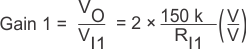Equation 8.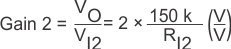If summing left and right inputs with a gain of 1 V/V, use RI1= RI2= 300 kΩ.

This configuration will use resistor values of RI1 = 3 MΩ, and RI2 = 150 kΩ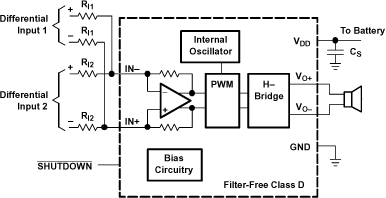Figure 37. Application Schematic With TPA2005D1 Summing Two Differential Inputs

#### 9.4.1.2 Summing a Differential Input Signal and a Single-Ended Input Signal

Figure 38 shows how to sum a differential input signal and a single-ended input signal. Ground noise can couple in through IN+ with this method. It is better to use differential inputs. The corner frequency of the single-ended input is set by CI2, shown in equation Equation 11. To assure that each input is balanced, the single-ended input must be driven by a low-impedance source even if the input is not in use.

Equation 9.Equation 10.Equation 11.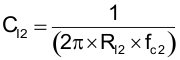If summing a ring tone and a phone signal, the phone signal should use a differential input signal while the ring tone might be limited to a single-ended signal. Phone gain is set at gain 1 = 0.1 V/V, and the ring-tone gain is set to gain 2 = 2 V/V, The resistor values are RI1 = 3 MΩ and RI2 = 150 kΩ.

The high pass corner frequency of the single-ended input is set by CI2. If the desired corner frequency is less than 20 Hz.

Equation 12.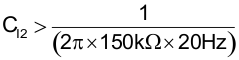Equation 13.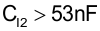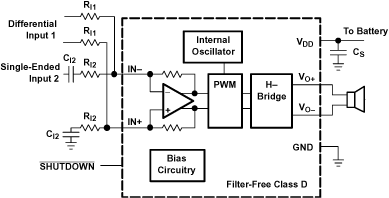Figure 38. Application Schematic With TPA2005D1 Summing Differential Input and
Single-Ended Input Signals

#### 9.4.1.3 Summing Two Single-Ended Input Signals

Four resistors and three capacitors are needed for summing single-ended input signals. The gain and corner frequencies (fc1 and fc2) for each input source can be set independently (see equations through Equation 17, and Figure 39). Resistor, RP, and capacitor, CP, are needed on the IN+ terminal to match the impedance on the IN- terminal. The single-ended inputs must be driven by low impedance sources even if one of the inputs is not outputting an ac signal.

Equation 14.Equation 15.Equation 16.Equation 17.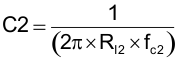Equation 18.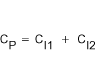Equation 19.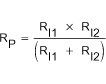Figure 39. Application Schematic With TPA2005D1 Summing Two Single-Ended Inputs

#### 9.4.2 Shutdown Mode

The TPA2005D1 can be put in shutdown mode when asserting SHUTDOWN pin to a logic LOW. While in shutdown mode, the device output stage is turned off and set into high impedance, making the current consumption very low. The device exits shutdown mode when a HIGH logic level is applied to SHUTDOWN pin.